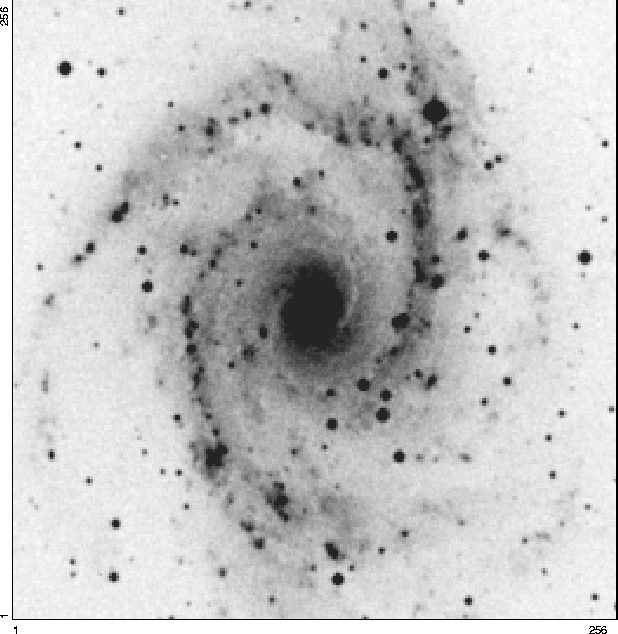Next: Visualisation of the first Up: The Wavelet Transform Previous: The Reconstruction

Visualization of the Wavelet TransformWe have seen that the wavelet transform furnishes a number of data which depends on the algorithm used. We distinguish three classes of algorithms:

• those which do not reduce the sampling. The number of wavelet coefficients is equal to the number of pixels of the image multiplied by the number of scales. This is the case if we use the à trous algorithm.
• those which furnish a pyramidal set of data
• those which furnish an image
In the following, we present how the galaxy (figure 14.12) can be represented in the wavelet space.

Petra Nass
1999-06-15